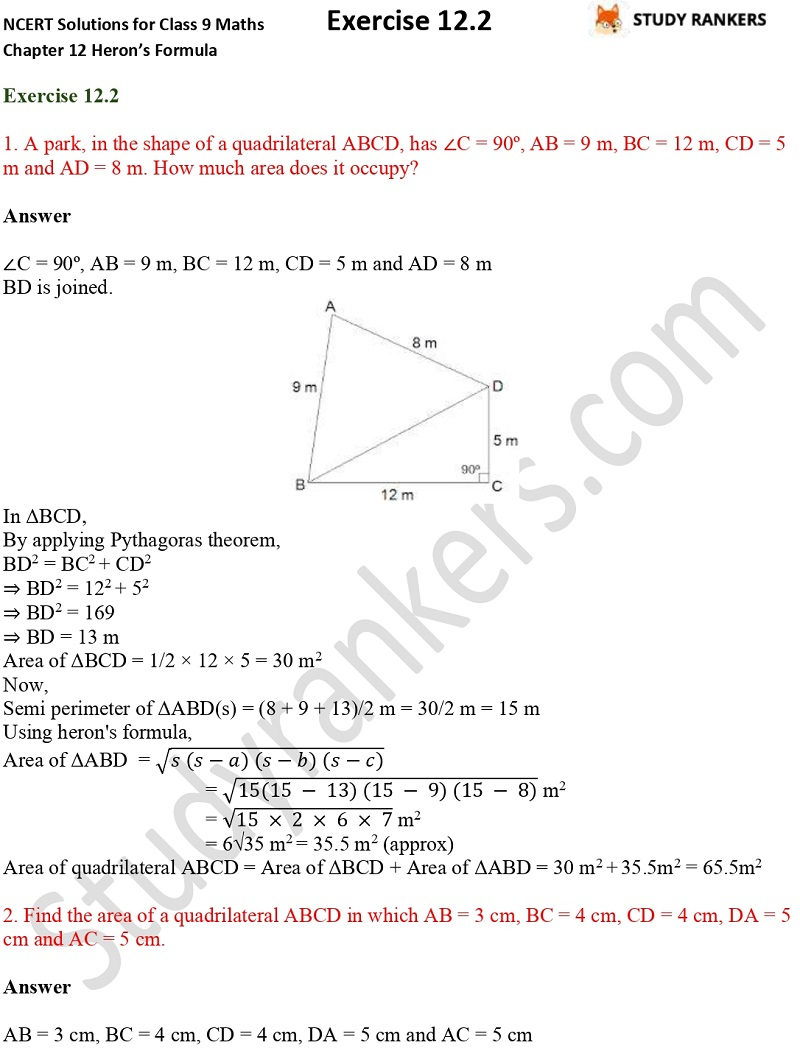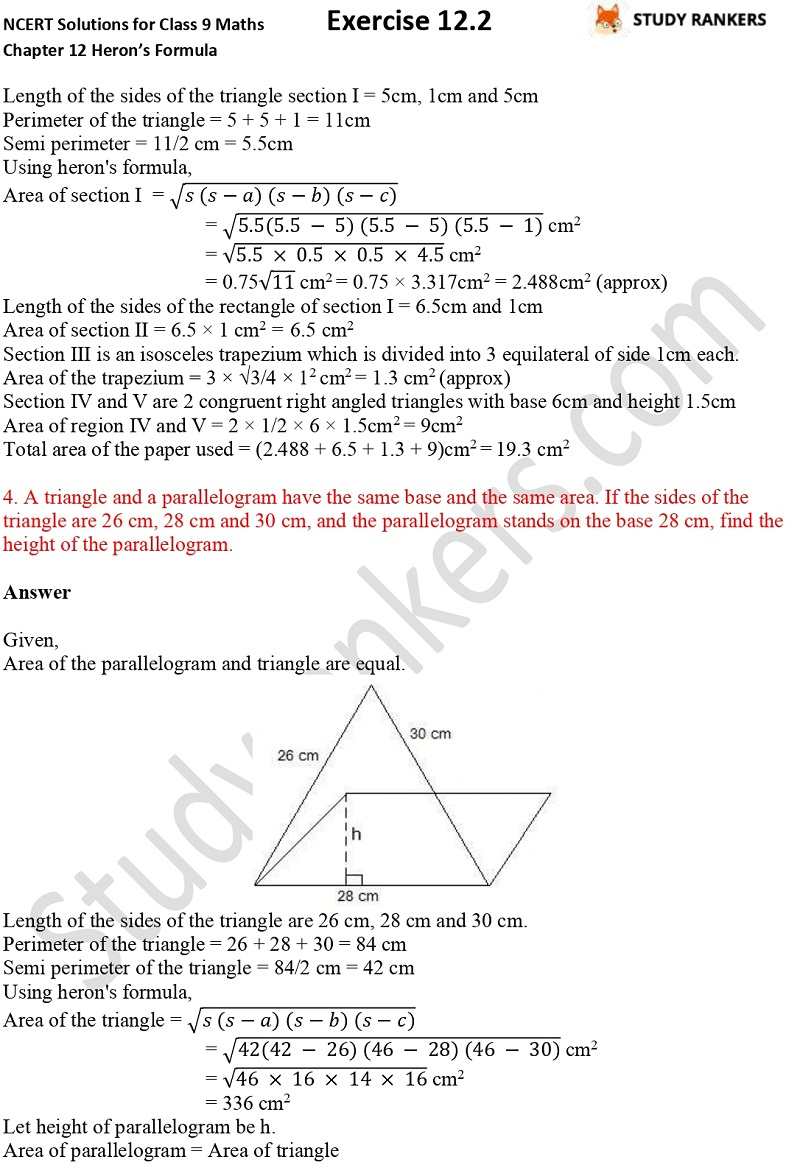>

## NCERT Solutions for Class 9 Maths Chapter 12 Heron's Formula Exercise 12.2

Class 9 Maths Chapter 12 Heron's Formula Exercise 12.2 NCERT Solutions is provided on this page that will help students in completing their homework and understanding the basics. Class 9 Maths NCERT Solutions can be useful for boosting your marks in the examinations as we have prepared accurate and detailed solutions so you don't need to waste your precious time wandering for answers. The NCERT questions and answers are updated as per the latest syllabus released by CBSE.

There are total 9 questions in Exercise 12.2 which are about finding the area of field through Heron's Formula.X